Thermodynamics and Propulsion

15.6 Muddiest points on Chapter 15

MP 15..1   Why is there 3.76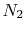?

This is to represent the components other than oxygen that are in air. From SB&VW, page 525, The assumption that air is 21% oxygen and 79% nitrogen by volume leads to the conclusion that for each mole of oxygen,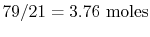of nitrogen are involved.''

MP 15..2   What is the most effective way to solve for the number of moles in the reactions?

What we are doing is basically counting atoms on both sides of the reactantsproducts statement. See Section 14.2 of SB&VW for a description of the combustion process and for going from ratios in terms of moles to ratios in terms of mass.

MP 15..3   Do we always assume 100% complete combustion? How good an approximation is this?

In the problems we do, we will only consider 100% combustion. It is a good approximation for the range of problems that we address.

MP 15..4   Is the enthalpy of formation equal to the heat transfer out of the combustion during the formation reaction?

As defined, the enthalpy of formation relates to a process in which the initial and final states are at the same temperature. If there is combustion in between, heat will have to be removed for this condition to occur. The enthalpy of formation is equal to the negative of the magnitude of the heat outflow. If we consider the combustion as occurring in a control volume, then per kmole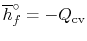, where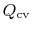is the heat transfer out of the control volume per kmole. This is not in accord with our convention, and if you please feel free to transform it back into the notation we have used before. (I find that if I do this there are too many minus signs to keep track of easily.)

MP 15..5   Are the enthalpies ofand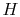(monoatomic hydrogen) both zero at?

The enthalpies of the elements are taken as zero atand. In some cases there are more than one form of the element. In that case the form chosen to have the value of zero is that which is chemically stable at the reference state. The other forms then have an enthalpy which is consistent with the reaction that produces this form of the element. For hydrogenhas zero enthalpy at the reference conditions andhas an enthalpy of(see Table A.8 in SB&VW), consistent with the idea that energy has to be supplied to break the molecule apart.

MP 15..6   When doing a cycle analysis, do we have to consider combustion products and their effect on specific heat ratio (is not 1.4)?

The specific heat ratio does depend on combustion products but the effect is not large because the fuel air ratio is small. For example, for conditions of fuel air ratio 0.034, the specific heat ratio at room temperature is about 1.38. A larger variation encountered in practice is with temperature; for a temperature ofthe specific heat ratio of pure air is 1.3.

MP 15..7   Does adiabatic flame temperature'' assume 100% combustion?

Yes. This is the maximum temperature that could be produced. Incomplete combustion will lower the temperature, as will heat transfer out of the combustion region.

MP 15..8   What part of the computation for adiabatic flame temperature involves iteration?

If the specific heat is not a simple analytic function of temperature (i.e., suppose it is known only in tabular form), we cannot get a closed form solution for the adiabatic flame temperature. We can, however, readily solve the enthalpy balance (SFEE) numerically (this is where the iteration comes in) to find at what temperature the products have to come out to have the same enthalpy (including the enthalpy of formation) as the reactants. We did not do this calculation yet, but we will do it to show what the iteration is all about.

Remember that the assumption of constant specific heat is just that, an assumption. While this is an excellent assumption for many practical problems, if the precision of the answer needed is very high, or if the range of temperatures is large (see Figure 5.11 in SB&VW), then we cannot assume constant specific heat.

UnifiedTP